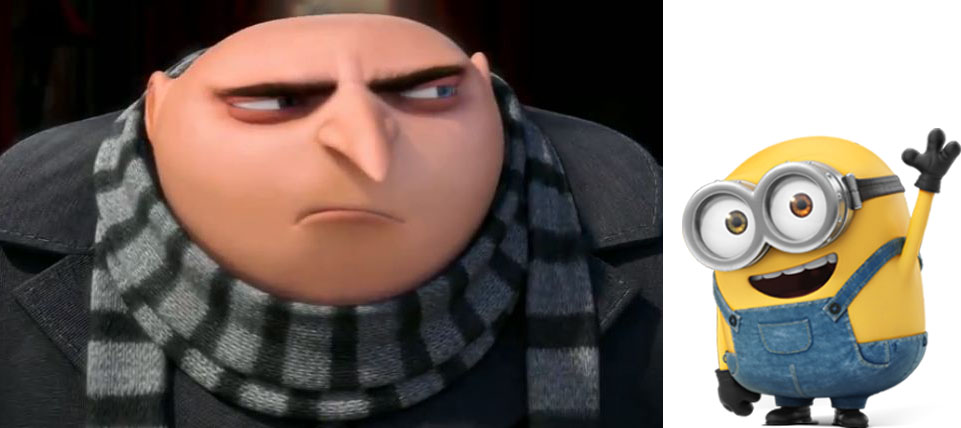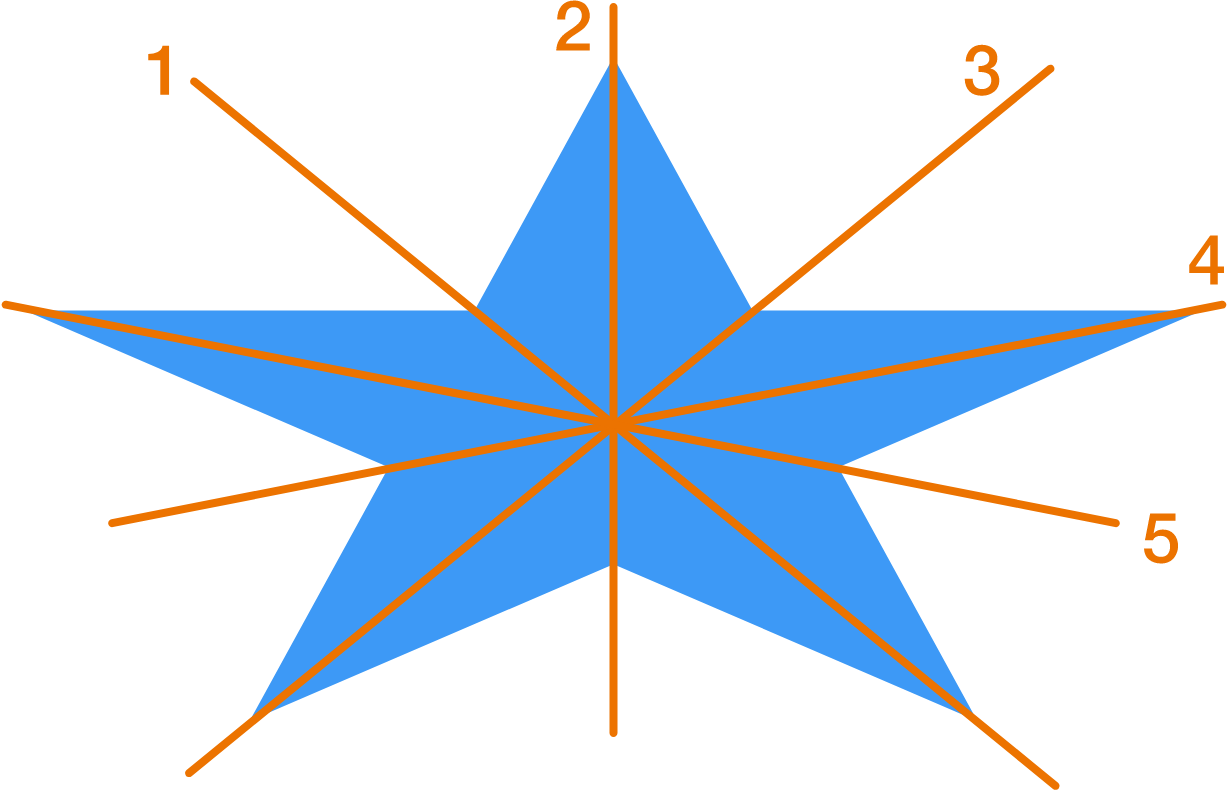# Evil Symmetry

Geometry Level 2Bob the minion is learning symmetry because he wants to become the evilest supervillain ever. Gru hands him the following figure as an exercise and asks him to mark all the lines of symmetry.

A Somewhat Squashed Star:Bob draws the following lines; however he is not sure if he is completely correct. Please help him.Which of these lines are not lines of symmetry for the given figure? Find the sum of all the incorrect lines.

• As an explicit example, if you think lines $2$ and $3$ are not lines of symmetry, then your answer is $2 + 3 = 5$.
×